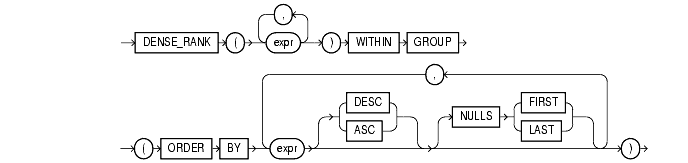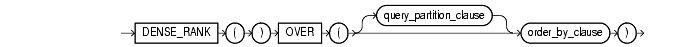Functions, 35 of 166

## DENSE_RANK

#### Aggregate Syntax

dense_rank_aggregate::=Text description of `dense_rank_aggregate`

#### Analytic Syntax

dense_rank_analytic::=Text description of `dense_rank_analytic`

#### Purpose

The `DENSE_RANK` function computes the rank of a row in an ordered group of rows. The ranks are consecutive integers beginning with 1. The largest rank value is the number of unique values returned by the query. Rank values are not skipped in the event of ties. Rows with equal values for the ranking criteria receive the same rank.

• As an aggregate function, `DENSE_RANK` calculates the dense rank of a hypothetical row identified by the arguments of the function with respect to a given sort specification. The arguments of the function must all evaluate to constant expressions within each aggregate group, because they identify a single row within each group. The constant argument expressions and the expressions in the order_by_clause of the aggregate match by position. Therefore, the number of arguments must be the same and types must be compatible.

• As an analytic function, `DENSE_RANK` computes the rank of each row returned from a query with respect to the other rows, based on the values of the value_exprs in the order_by_clause.

#### Aggregate Example

The following example computes the ranking of a hypothetical employee with the salary \$15,500 and a commission of 5% in the sample table `oe.employees`:

```SELECT DENSE_RANK(15500, .05) WITHIN GROUP
(ORDER BY salary DESC, commission_pct) "Dense Rank"
FROM employees;

Dense Rank
-------------------
3
```

#### Analytic Example

The following statement selects the department name, employee name, and salary of all employees who work in the `HUMAN` `RESOURCES` or `PURCHASING` department, and then computes a rank for each unique salary in each of the two departments. The salaries that are equal receive the same rank. Compare this example with the example for RANK.

```SELECT d.department_name, e.last_name, e.salary, DENSE_RANK()
OVER (PARTITION BY e.department_id ORDER BY e.salary) as drank
FROM employees e, departments d
WHERE e.department_id = d.department_id
AND d.department_id IN ('30', '40');

DEPARTMENT_NAME         LAST_NAME              SALARY      DRANK
----------------------- ------------------ ---------- ----------